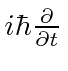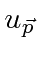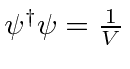## Solution of Dirac Equation for a Free Particle

As with the Schrödinger equation, the simplest solutions of the Dirac equation are those for a free particle. They are also quite important to understand. We will find that each component of the Dirac spinor represents a state of a free particle at rest that we can interpret fairly easily.

We can show that a free particle solution can be written as a constant spinor times the usual free particle exponential. Start from the Dirac equation and attempt to develop an equation to show that each component has the free particle exponential. We will do this by making a second order differential equation, which turns out to be the Klein-Gordon equation.The free electron solutions all satisfy the wave equation.Because we have eliminated thematrices from the equation, this is an equation for each component of the Dirac spinor. Each component satisfies the wave (Klein-Gordon) equation and a solution can be written as a constant spinor times the usual exponential representing a wave.Plugging this into the equation, we get a relation between the momentum and the energy.Note that the momentum operator is clearly stilland the energy operator is still.

There is no coupling between the different components in this equation, but, we will see that (unlike the equation differentiated again) the Dirac equation will give us relations between the components of the constant spinor. Again, the solution can be written as a constant spinor, which may depend on momentum, times the exponential.We should normalize the state if we want to describe one particle per unit volume:. We haven't learned much about what each component represents yet. We also have the plus or minus in the relationto deal with. The solutions for a free particle at rest will tell us more about what the different components mean.

Subsections
Jim Branson 2013-04-22